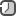### Random Numbers in R

Random numbers are critical to many analyses, and consequently R has a wide variety of functions for sampling from different distributions.

A `sample` function is important workhorse function for generating random values, and its behavior has a few quirks, so it’s worth getting to know it more thoroughly. If you just pass it a number, n, it will return a permutation of the natural numbers from 1 to n

```sample(7)
 1 2 5 7 4 6 3
```

If you give it a second value, it will return that many random numbers between 1 and n

```sample(7, 5)
 7 2 3 1 5
```

Notice that all those random numbers are different. By default, `sample` samples without replacement. That is, each value can only appear once. To allow sampling with replacement, pass `replace = TRUE`

```sample(7, 10, replace = TRUE)
 4 6 1 7 5 3 6 7 4 2
```

Also, you can select n items from a vector

```#sample(vec, n)
sample(world.series\$year, 10)
 1906 1963 1966 1928 1905 1924 1961 1959 1927 1934
```

The following example generates a random sequence of 10 simulated flips of a coin

```sample(c("H","T"), 10, replace=TRUE)
 "H" "H" "H" "T" "T" "H" "T" "H" "H" "T"
```

By default, sample will choose equally among the set elements and so the probability of selecting either H or T is 0.5. With a Bernoulli trial, the probability p of success is not necessarily 0.5. You can bias the sample by using the `prob` argument of `sample`. This argument is a vector of probabilities, one for each set element.

Suppose we want to generate 10 Bernoulli trials with a probability of success p = 0.8. We set the probability of H to be 0.2 and the probability of T to 0.8

```sample(c("H","T"), 10, replace=TRUE, prob=c(0.2,0.8))
 "T" "T" "T" "T" "T" "H" "H" "T" "H" "T"
```